Creating an Algorithmic Trading Prototyping Environment with Jupyter Notebooks and Plotly

In this tutorial we will be setting up a prototyping environment using jupyter notebook to analyse our data in a reproducible manner

In the previous article we installed Python and set up our virtual environment. We then used pandas-datareader directly in the python terminal in order to import some equities OHLC data and plot five years of the adjusted close price. This was accomplished in a few lines of code. However, once we closed the terminal we lost all the data. In this tutorial we will be setting up a prototyping environment using Jupyter notebook to analyse our data in a reproducible manner. The libraries we are using for this tutorial are:

• Matplotlib v3.5
• Pandas v1.4
• jupyter v1.0
• plotly v5.6
• ipykernel v6.4

For the purpose of this tutorial we created a Python environment using Python v3.8

Anaconda comes with Jupyter notebook installed and ready to go. Once you are inside the base environment you can simply type jupyter notebook from within any directory and a window will open in your browser showing you all the files and folders located in that directory. From this page you can create a notebook by clicking "new".

This will run from your base anaconda environment and therefore have access to all the python packages installed in that environment. However, you will recall in the previous article that we created a virtual environment into which we installed Matplotlib, Pandas and pandas-datareader. If you were to try and import pandas-datareader into a notebook from the base anaconda environment you would get the following error ModuleNotFoundError. This is because, by design, the base anaconda environment doesn't have access to the libraries installed in the virtual environment.

## Setting up Jupyter Notebook with ipykernel

One of the simplest ways to access the libraries in your virtual environment through Jupyter notebook is to use ipykernel. This package is installed directly into your virtual environment and will enable you to choose the kernel approriate to your virtual environment from within the Jupyter notebook interface. Let's have a look at one of the easiest ways to accomplish this.

We start by creating a virtual environment, activating it and installing our required libraries. If you have been following along with this article series you could simply activate the py3.8 environment we created last time. If not you will need to create a virtual environment an install Matplotlib, Pandas and pandas-datareader.

(base)$conda activate py3.8  Once inside the virtual environment you need to install ipykernel (py3.8)$ conda install ipykernel


Now all you need to do is register the kernel specification with Jupyter. You can name your kernel anything you like after the --name= parameter.

(py3.8)$ipython kernel install --user --name=py3.8  Now that we have a working kernel for our virtual environment all we need to do is open Jupyter notebook from our anaconda base environment. Then we can create a new notebook specifying the kernel you have just created. Now when you call import pandas_datareader.data as web you won't get an error. Open a new terminal window in the base anaconda environment (Hint: Check that (base) is displayed in the terminal window before the user information.) Now type jupyter notebook to open jupyter in your browser. Once open click new and select your new kernel from the dropdown.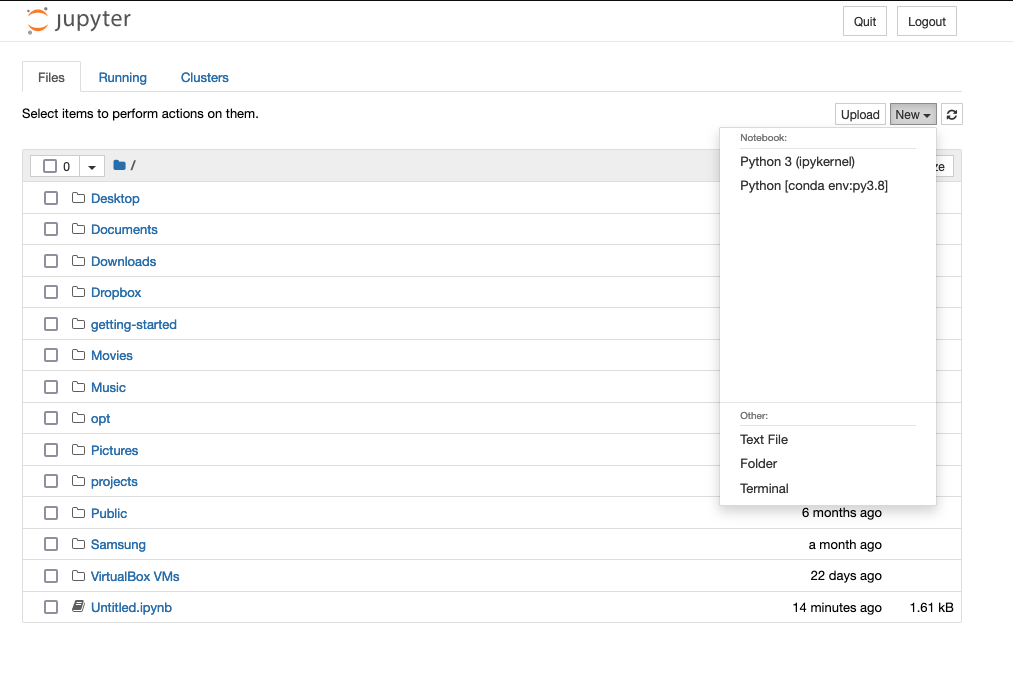Accessing the new kernel in Jupyter Notebook ## Importing and plotting data We will begin by recreating our quick analysis from the previously article, except this time all our work will be incorported into a Jupyter notebook which we can access repeatedly and build upon over time. A full overview of the Jupyter commands is outside the scope of this article as we will mainly be focusing on creating financial visualizations. There are some great tutorials available to discover the full potential of the software dataquest and datacamp are among them. Briefly, the most frequently used commands are: • Enter edit mode with enter • Enter command mode with esc • Once in command mode (after pressing esc) • M will transform a cell to Markdown so you can add text • Y will transform a cell to code • A and B will add a new cell above or below • Move up and down cells with Up and Down arrow keys • DD will delete the cell • H will open the keyboard shortcuts menu We start by importing our libraries: type the following into the first cell and press shift+enter to run the cell and create a new one underneath import matplotlib.pyplot as plt import pandas as pd import pandas_datareader.data as web from datetime import datetime as dt  We now define our date range and import our data: start = dt(2016, 11,1) end = dt(2021, 11, 1) aapl = web.DataReader("AAPl", "yahoo", start, end)  To view the first few lines of our DataFrame type aapl.head().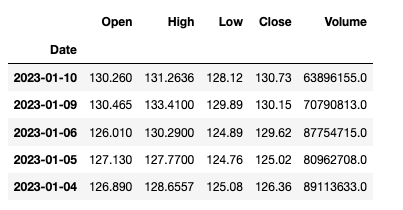First rows of aapl DataFrame Now we simply plot as before by typing aapl.plot(y="Adj Close") into a new cell and pressing shift+enter.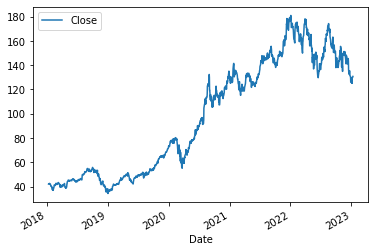5 Years of apple adjusted close price in Jupyter notebook ## Plotting Candlesticks in Jupyter Candlestick charts are thought to date back to Japan in the late 1800s. They are composed of the real body, the area between the open and close price and the wicks or shadows, the excursions above and below the real body that illustrate the highest and lowest prices of an asset in the time period represented. If the asset closes at a price higher than the opening price the body is usually unfilled or hollow. If the asset closes lower than it opened the body of the candlestick may be filled or solid. The colour of the candlestick is representaive of the price movement for the period of time represented. Black or red candlesticks indicate that the closing price is lower than the previous time point and a green or white candle means that the closing price is higher than the previous time point. In practice the color and fill of the candlestick can be designated by the user.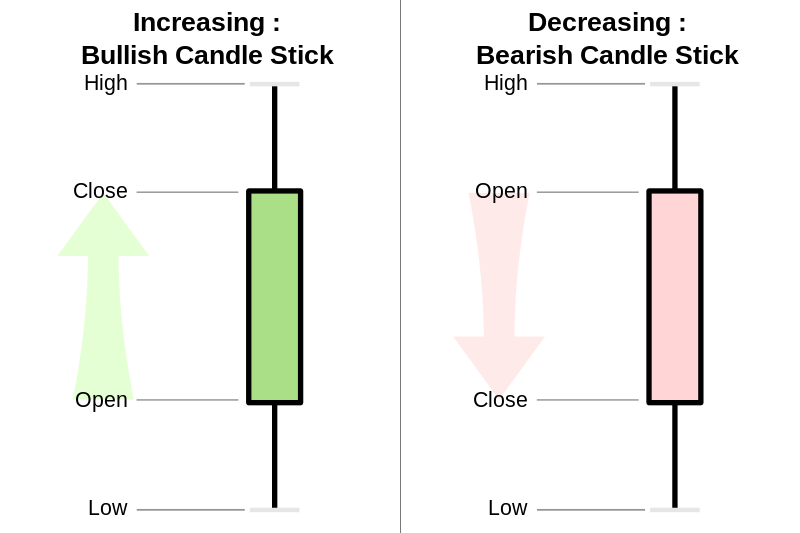Candlestick chart scheme courtesy of Wikipedia There are several different ways to create candlestick plots in Python. You can create your own script in Matplotlib using boxplots but there are also a number of different open source libraries such as mplfinance, bqplot, Bokeh and Plotly. There is an excellent article offering an overview of each here. In this article we will be using Plotly to create our candlestick plots. The Plotly Python graphing library offers over 40 different chart types for statistical, financial, scientific and 3-dimensional uses. Early versions of this charting library required users to sign up for access with an API key each time they created a plot. There was also the option to publish the images to an online repository of charts by choosing between online and offline modes of operation. Since version four of the software it is no longer necessary to have an account or internet connection and no payment is required to use Plotly.py. The latest version of the software at time of writing is version 5.6.0. In order to use Plotly we will need to install it into our virtual environment. In the terminal inside the same virtual environment that you used to create your kernel install Plotly through conda. (py3.8)$ conda install -c plotly plotly


Once the install is complete you need to restart the kernel in your Jupyter notebook. In the menu select Kernel then Restart and Run all from the dropdown. This will re run all the cells in your notebook and refresh the Kernel to include access to Plotly.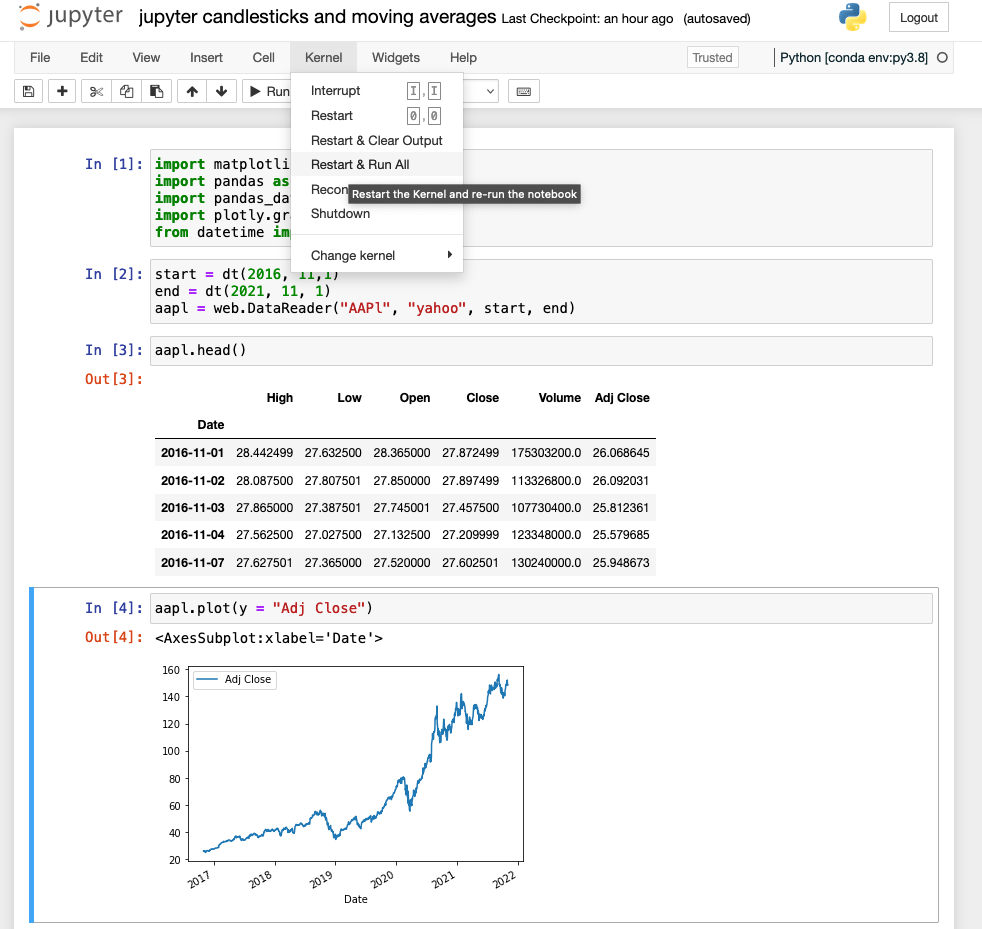Restarting the Kernel in Jupyter notebook

To simplify the images we will begin by looking at a month of OHLC data. In a new cell in your notebook enter the following code to create a new DataFrame "goog" with a month of OHLC Google data from Yahoo.

start = dt(2021, 10,1)
end = dt(2021, 11, 1)
goog = web.DataReader("GOOG", "yahoo", start, end)


You can check your DataFrame by typing goog.head(). We now need to import Plotly into our notebook. Best practice is to place all imports at the top of your code, in alphabetical order. This ensures that when anyone is reviewing your code they can see what libraries have been added and when a method is called from any of the libraries it is easy to determine where that method originated. You can also alias your imports as we have here. This means that whenever you want to use a method from the libraries you don't need to type plotly.graph_objects you can simply type the alias, in this case, "go". Add import plotly.graph_objects as go to the first cell in your notebook underneath the pandas_datareader import.

Plotly comes with an interactive candlestick figure prebuilt. All you need to do is define your data and call the figure. The Candlestick method requires you to specify your x axis data, the open, high, low and close price in order to generate the figure. We will also add a name to our plot so that if we choose to add additional trendlines to our figure this name will appear in the legend.

# define the data
candlestick = go.Candlestick(
x=goog.index,
open=goog['Open'],
high=goog['High'],
low=goog['Low'],
close=goog['Close'],
name="OHLC"
)

# create the figure
fig = go.Figure(data=[candlestick])

# plot the figure
fig.show()


The figure generated is interactive. You can hover over any of the candlesticks and see the data that created it. There is also a range slider at the bottom of the figure allowing you to zoom into a specific data range. This can be disabled by adding the following line above fig.show(): fig.update_layout(xaxis_rangeslider_visible=False)

## Adding Trendlines to Plotly Candlestick Charts

The Figure() method takes a keyword data which accepts a list. This allows us to add trendlines to our graph by defining additional variables containing the Scatter method. Let's see this in action by overlaying a five day moving average to our candlestick plot. To begin we first need to calculate the moving average, this can be done by chaining the Pandas rolling() and mean() methods. In a new cell we will add a Series to our "goog" DataFrame containing the value of the five day moving average at each time point.

  goog['MA5'] = goog.Close.rolling(5).mean()


The commmand goog.head() shows the new column with the value appearing in the fifth row as expected.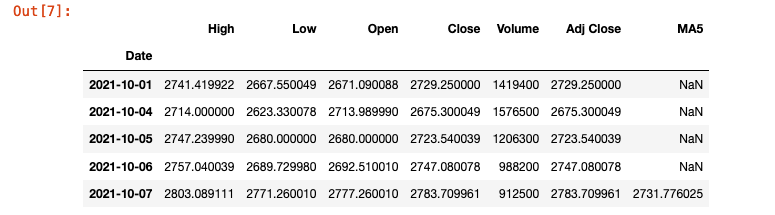Five day moving average in DataFrame

Now we can create our scatter object by defining our x and y data points. We can create a trendline rather than markers by using the keywordline and control the color and width of the line.

  scatter = go.Scatter(
x=goog.index,
y=goog.MA5,
line=dict(color='black', width=1),
name="5 day MA"
)


Once we have defined our data we can add it to our plot by adding the scatter variable to the list in the data keyword.

  fig = go.Figure(data=[candlestick, scatter])
fig.show()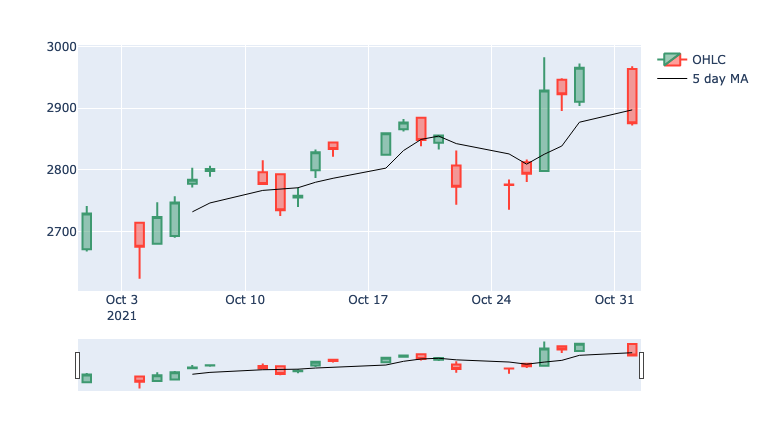Candlestick chart with five day moving average trendline

## Adding Volume to Plotly Candlestick Charts

A common modification to a candlestick chart is the addition of volume. This can be accomplished in two ways; as an overlay on the existing chart with a secondary axis or underneath the original candlestick chart. Both options require us to make use of the Plotly make_subpplots class. The first thing we need to do is to import the class at the top of our notebook. To keep with best practice, underneath the plotly.graph_objects import add the following line: from plotly.subplots import make_subplots. Now we can create a figure with secondary axis for the volume. First we create the secondary axis then we add a trace for the candlestick OHLC data and a scatter for the five day moving average. This can be done by invoking the candlestick and scatter variables we created earlier. Then we add a trace for a bar chart containing the volume data. We can control the transparency of the bar chart with the opacity keyword and the colour using the marker_colour keyword. Finally we turn off the gridlines for the secondary axis to avoid confusion in the final chart and display the figure.

  # create a figure with a secondary axis
fig = make_subplots(specs=[[{"secondary_y": True}]])

candlestick,
secondary_y=False
)

scatter,
secondary_y=False
)

go.Bar(x=goog.index, y=goog['Volume'], opacity=0.1, marker_color='blue', name="volume"),
secondary_y=True
)
fig.layout.yaxis2.showgrid=False
fig.show()


If you would prefer to keep the volume as a seperate chart this can also be very easily created using Plotly. All we need to do is add an additional plot in the call to make_subplots and define the position of each trace within the subplots. We will begin by defining our subplot using rows and columns, in this case we want two figures on top of each other so we have one column and two rows. We share the x axis as the time series dates are the common factor between the two plots. We give an additional vertical space of 0.1 to accommodate our subplot titles, which we then define. Finally we specify how high we would like our rows to be in relation to each other. The total value of the row heights will be normalised so that they sum to 1. After defining our subplots we simply add each trace as we did previously specifying the position of each trace within the subplots. We then disable the range slider to avoid confusion and display the final figure.

  # Create subplots
fig = make_subplots(
rows=2, cols=1,
shared_xaxes=True,
vertical_spacing=0.1,
subplot_titles=('OHLC', 'Volume'),
row_heights=[0.7, 0.3]
)

# Plot candlestick on 1st row
candlestick,
row=1, col=1
)
# Plot scatter on 1st row
scatter,
row=1, col=1
)

# Plot Bar trace for volumes on 2nd row without legend
go.Bar(
x=goog.index,
y=goog['Volume'],
opacity=0.1,
marker_color='blue',
showlegend=False
),
row=2, col=1
)

# Do not show OHLC's rangeslider plot
fig.update(layout_xaxis_rangeslider_visible=False)
fig.show()